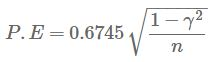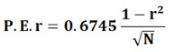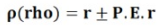Courses

# Probable Error - Correlation & Regression, Business Mathematics & Statistics B Com Notes | EduRev

## B Com : Probable Error - Correlation & Regression, Business Mathematics & Statistics B Com Notes | EduRev

The document Probable Error - Correlation & Regression, Business Mathematics & Statistics B Com Notes | EduRev is a part of the B Com Course Business Mathematics and Statistics.
All you need of B Com at this link: B Com

Probable Error
The term probable error is seen only in older statistics literature where it used to denote the product of the standard error and a constant factor 0.6745. Thus,
P.E = 0.6745(S.E)
Here P.E and S.E stand for the probable error and the standard error, respectively. By using the above formula the probable error of any statistic may be determined if we substitute the standard error of that statistic. Hence, for example, the probable error of the correlation coefficient would beDefinition: The Probable Error of Correlation Coefficient helps in determining the accuracy and reliability of the value of the coefficient that in so far depends on the random sampling.
In other words, the probable error (P.E.) is the value which is added or subtracted from the coefficient of correlation (r) to get the upper limit and the lower limit respectively, within which the value of the correlation expectedly lies.
The probable error of correlation coefficient can be obtained by applying the following formula:r = coefficient of correlation
N = number of observations

• There is no correlation between the variables if the value of ‘r’ is less than P.E. This shows that the coefficient of correlation is not at all significant.
• The correlation is said to be certain when the value of ‘r’ is six times more than the probable error; this shows that the value of ‘r’ is significant.
• By adding and subtracting the value of P.E from the value of ‘r,’ we get the upper limit and the lower limit, respectively within which the correlation of coefficient is expected to lie. Symbolically, it can be expressedwhere rho denotes the correlation in a population
The probable Error can be used only when the following three conditions are fulfilled:

1. The data must approximate to the bell-shaped curve, i.e. a normal frequency curve.
2. The Probable error computed from the statistical measure must have been taken from the sample.
3. The sample items must be selected in an unbiased manner and must be independent of each other.

Thus, the probable error is calculated to check the reliability of the value of coefficient calculated from the random sampling.

Offer running on EduRev: Apply code STAYHOME200 to get INR 200 off on our premium plan EduRev Infinity!

122 videos|142 docs

,

,

,

,

,

,

,

,

,

,

,

,

,

,

,

,

,

,

,

,

,

,

,

,

;